# How to Calculate Net Work Hours Between Two DatesCounting the number of hours between dates and times in Excel is normally a straightforward process. Since Excel stores dates as decimal numbers, you can just subtract the two to get your result. But when you are working with business hours, like for time sheets or hours worked, you need to take weekends and holidays into account. Excel has a function called NETWORKDAYS, but this only works with complete days. To calculate the Net Work Hours between two dates, we need to build our own formula. Here’s how…

## Details of the Net Work Hours Formula

A lot of assumptions have to be made about any formula that works with dates and times. Definitions of terms like work day, weekend, and holiday can vary, so this is a summary of the assumptions of this net work hours formula:

• The eligible work hours in a given work day start and end at the same time each work day.
• Weekend days do not count as work days. The weekend is defined as Saturday and Sunday of each week (look ahead to Non-Standard Weekends with NETWORKDAYS.INTL to change this).
• Holidays, as listed, do not count as work days.
• Partial work days at the start and end of a date period are included for their fraction of hours.
• If the start time of the work day is later than the end time of the work day, the formula nets zero.
• If the start date and time is later than the end date and time, the formula nets zero.

There are modifications available to the above rules later in this tutorial:

If your definition of weekend is different than above and you have Excel 2007 or newer, you can look ahead to Non-Standard Weekends with NETWORKDAYS.INTL to change this.

If you want to include all 24 hours of each work day rather than a specified range of work hours, you can look ahead to 24 Hour Net Workday Hours Variation to tally the total number of hours each work day.

## Setting Up the Variables for Net Work Hours

In order to calculate the net work hours between two dates, we need to have some information to start with. Here are the variables necessary to make the net work hours formula work.

\$Start_Time – This is the time that the work day starts, as an Excel-formatted time. (e.g. 9:00AM) No date should be attached. The variable is marked with a dollar sign because you will probably want to anchor this cell reference in the formula.

\$End_Time – This is the time that the work day ends, as an Excel-formatted time. (e.g. 5:00PM) No date should be attached. The variable is marked with a dollar sign because you will probably want to anchor this cell reference in the formula.

Start_Date – This is the starting date and time for your period, as an Excel-formatted date and time (e.g. 12/30/2013 11:00AM)

End_Date – This is the ending date and time for your period, as an Excel-formatted date and time (e.g. 1/14/2014 4:00PM)

HolidayList – This is a named range containing a list of days that are to be considered holidays and not counted as work days, even if they are not on the weekend. (e.g. New Year’s Day – 1/01/2013) It is usually located elsewhere on the worksheet.

### Setting Up the HolidayList Named Range

The function NETWORKDAYS takes an array input called [holidays] that lists all the non-weekend days that should be excluded from the calculation. This means that we could give the function a series of dates in brackets, but they would need to be formatted in Excel date format. This means that they need to be converted using a DATE function. (See the Definitive Guide to Using Dates and Times in Excel for an explanation.) An easier approach is to build a named range.

In a separate worksheet tab, or in an un-used portion of your current spreadsheet, make a column list of the dates you want to include as holidays in Excel date-format. You can either use a DATE function to specify the year, month, and day for each, or let Excel auto-convert as you type.

When you finish your list, select the list (and maybe a few extra cells, if you plan to add to it later). The name of the first cell in the selection appears in the name box above the spreadsheet cells…With your list selected, select the cell name in the name box, type in “HolidayList“, and press ENTER.You have now named the selected cells as an array. It can be referred to in the net work hours formula as HolidayList instead of manually entering the range, and the NETWORKDAYS function can automatically exclude those dates from calculations.

## The Net Work Hours Formula

Here is the formula, in all it’s glory. Replace the variable names like \$Start_Time and HolidayList with your specific references. You can look to the sample file at the end of this post for an example of the formula in action.

```=IF(OR(\$End_Time<\$Start_Time,End_Date<Start_Date),0,
(NETWORKDAYS(Start_Date,End_Date,HolidayList)
-(NETWORKDAYS(Start_Date,Start_Date,HolidayList)
*IF(MOD(Start_Date,1)>\$End_Time,1,
(MAX(\$Start_Time,MOD(Start_Date,1))-\$Start_Time)
/(\$End_Time-\$Start_Time)))
-(NETWORKDAYS(End_Date,End_Date,HolidayList)
*IF(MOD(End_Date,1)<\$Start_Time,1,
(\$End_Time-MIN(\$End_Time,MOD(End_Date,1)))
/(\$End_Time-\$Start_Time))))
*(\$End_Time-\$Start_Time)*24)```

We’ll walk through how it works in a moment, but first, let’s look at a couple of variations that can solve different versions of the net work hours problem.

## Non-Standard Weekends with NETWORKDAYS.INTL

If you have Excel 2007 or newer, you can use a modified version of the NETWORKDAYS function called NETWORKDAYS.INTL to re-define what days are the “weekend” of each week. NETWORKDAYS.INTL takes an additional input called [weekend] that defines from a list which days are included in the weekend. The syntax for NETWORKDAYS and NETWORKDAYS.INTL are as follows:

`=NETWORKDAYS(start_date, end_date, [holidays])`
`=NETWORKDAYS.INTL(start_date, end_date, [weekend], [holidays])`

Note the additional input [weekend]. The [weekend] input has the following input options:If you just enter 1, NETWORKDAYS.INTL behaves identically to NETWORKDAYS. Other options give you additional flexibility on your definition of “weekend”.

For example, if you want to define the weekend as Monday and Tuesday, the net work hours formula becomes the following:

```=IF(OR(\$End_Time<\$Start_Time,End_Date<Start_Date),0,
(NETWORKDAYS.INTL(Start_Date,End_Date,3,HolidayList)
-(NETWORKDAYS.INTL(Start_Date,Start_Date,3,HolidayList)
*IF(MOD(Start_Date,1)>\$End_Time,1,
(MAX(\$Start_Time,MOD(Start_Date,1))-\$Start_Time)
/(\$End_Time-\$Start_Time)))
-(NETWORKDAYS.INTL(End_Date,End_Date,3,HolidayList)
*IF(MOD(End_Date,1)<\$Start_Time,1,
(\$End_Time-MIN(\$End_Time,MOD(End_Date,1)))
/(\$End_Time-\$Start_Time))))
*(\$End_Time-\$Start_Time)*24)```

Note the change to NETWORKDAYS.INTL and the use of 3 as the input for the new [weekend] term in the function.

## 24 Hour Net Workday Hours Variation

The above two versions operate on the assumption that there are defined start times and end times to each work day. Sometimes, though, we need to count all 24 hours of each eligible day. This is actually a simpler problem to solve, since we don’t need to look for ineligible hours in eligible work days. Therefore, the formula becomes:

```=IF(Start_Date>End_Date,0,(NETWORKDAYS(Start_Date,End_Date,HolidayList)
-NETWORKDAYS(Start_Date,Start_Date,HolidayList)*MOD(Start_Date,1)
-NETWORKDAYS(End_Date,End_Date,HolidayList)*(1-MOD(End_Date,1)))*24)```

If you need to use a non-standard weekend definition, you can replace the NETWORKDAYS functions in the formula with the NETWORKDAYS.INTL as shown in the Non-Standard Weekend section above.

## How the Net Work Hours Formula Works

There are a lot of moving parts to this formula, so it is useful to step through it procedurally. We’ll use an example case to walk through the logic of the formula step-by-step.

For this example, we’ll assume the following:

Start_Date is December 30, 2013 at 11:00 am.
End_Date is January 9, 2014 at 12:00 pm.
\$Start_Time is 9:00 AM.
\$End_Time is 5:00 PM.
HolidayList includes only January 1, 2014 (New Years Day).

### Step 1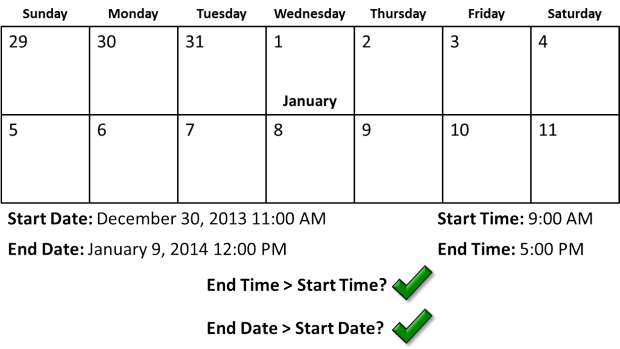Check if the dates or times are impossible (i.e. the period ends before it starts). If so, return zero, otherwise, continue to step 2.

### Step 2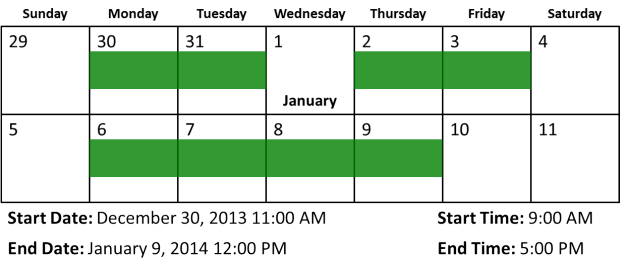Add the total number of days in the date series, minus weekends and holidays.

### Step 3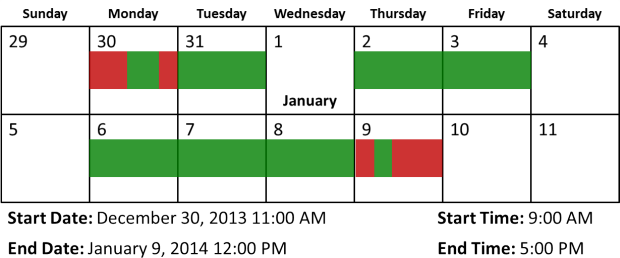Remove the greater of either the \$Start_Time or the Start_Date time on the Start_Date day. Remove the greater of the time after the \$End_Time or the time after the End_Date time on the End_Date day. (Not shown: If the Start_Date time starts after the \$End_Time, subtract an entire day. If the End_Date time comes before the \$Start_Time, subtract an entire day.)

### Step 4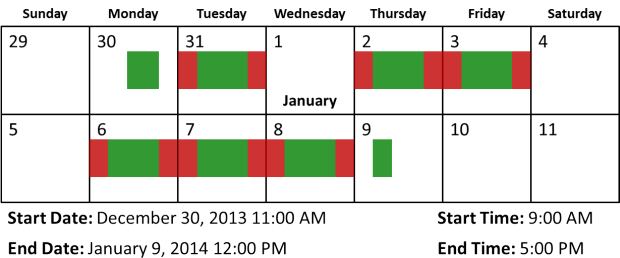For every work day between Start_Date and End_Date, count only the hours between \$Start_Time and \$End_Time.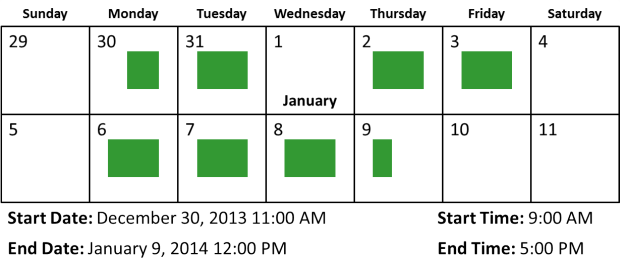This is the outcome of the subtractions in the formula.

### Step 5Add up the partial days in all the periods and multiply by 24 to calculate the total net work hours.Andrew Roberts has been solving business problems with Microsoft Excel for over a decade. Excel Tactics is dedicated to helping you master it. You can read more of his writing on his personal blog at NapkinMath.io.